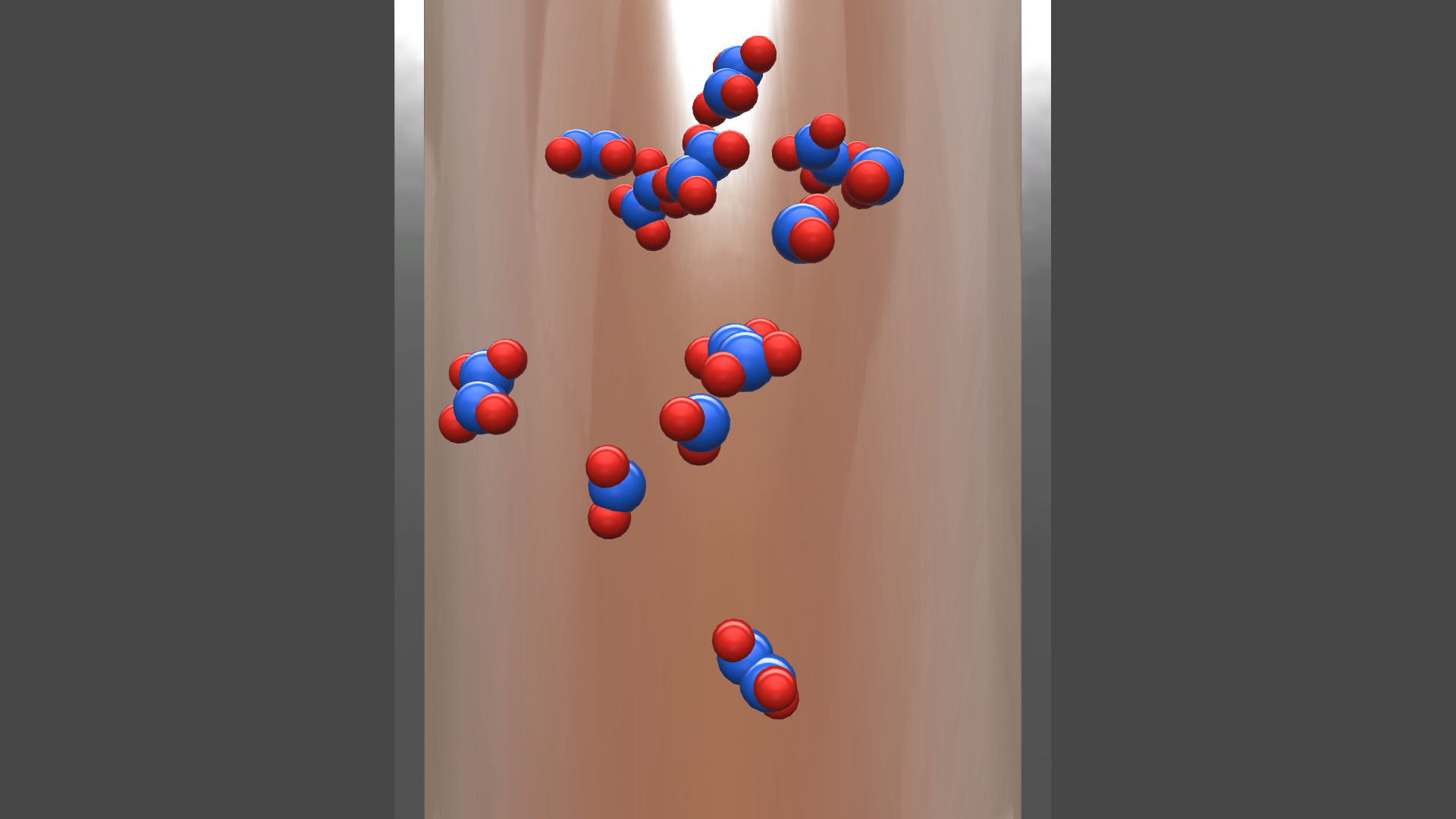Equilibrium

Learn about equlibrium and help a famous scientist to prevent a global famine by applying your knowledge to increase the yields of fertilizer for the crops.Try our lab safety simulation

Discover one of our 200+ learning simulations today

• Description
• Features

Equilibrium describes the state of a reversible reaction, in which the forward and backward reactions happen at equal rates. In this state, the concentrations of reactants and products are stable and do not change over time. Thus, there are no net changes in the concentrations of the reactant(s) and product(s). Such a state is known as dynamic equilibrium. It is a particular example of a system in a steady state.

Thermodynamics

In thermodynamics a closed system is in thermodynamic equilibrium when reactions occur at such rates that the composition of the mixture does not change with time. Reactions do in fact occur, sometimes vigorously, but to such an extent that changes in composition cannot be observed.

Help out a famous scientist

In chemistry there are several different types of equilibria: thermodynamic equilibrium, diffusive equilibrium, thermal equilibrium, and many more. In the Equilibrium Simulation, you will learn about the general chemical equilibrium and help a famous scientist to prevent a global famine. You will learn how to influence and predict the directionality of reversible reactions in the lab.

Will you be able to apply your knowledge to increase the yields of fertilizer for the crops?

Get started now

Learn about equlibrium and help a famous scientist to prevent a global famine by applying your knowledge to increase the yields of fertilizer for the crops.

Techniques in lab
Learning objectives

At the end of this simulation, you will be able to...

• Predict the directionality of reversible reactions according to Le Chatelier
• Calculate the equilibrium constant and reaction quotient
• Understand the Haber process
Simulation features

Length: 31
Accessibility mode: Available
Languages: French (Canada), English (United States)

NGSS

HS-PS1-6

IB

7.1 Equilibrium

AP

Chemistry 7.6 Properties of the Equilibrium Constant

Chemistry 7.1 Introduction to Equilibrium

Chemistry 7.2 Direction of Reversible Reactions

Chemistry 7.8 Representations of Equilibrium

Chemistry 7.9 Introduction to Le Châtelier's Principle

Chemistry 7.5 Magnitude of the Equilibrium Constant

Chemistry 7.7 Calculating Equilibrium Concentrations

Chemistry 7.3 Reaction Quotient and Equilibrium Constant

Chemistry 7.4 Calculating the Equilibrium Constant

Chemistry 7.10 Reaction Quotient and Le Châtelier's Principle